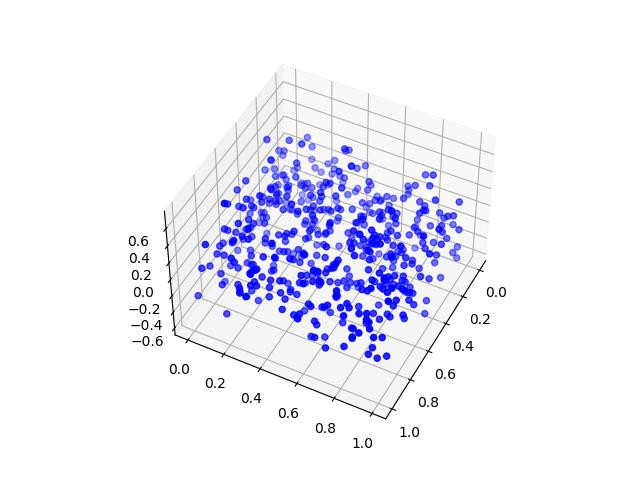#DAY 27
0
Software Development

## [Day 27] 從零開始學Python - 科學繪圖Matplotlib：畫著你，畫不出你的骨骼

``````pip install matplotlib
``````

``````import numpy as np
import matplotlib.pyplot as plt
``````

``````>>> mu, sigma = 0, 0.1
>>> s = np.random.normal(mu, sigma, 1000)
>>> plt.hist(s) # 繪製直方圖，預設分成10組
(array([ 10.,  32.,  91., 164., 238., 216., 147.,  71.,  25.,   6.]), array([-0.
30285466, -0.2403016 , -0.17774855, -0.1151955 , -0.05264245,
0.0099106 ,  0.07246365,  0.1350167 ,  0.19756975,  0.2601228 ,
0.32267585]), <BarContainer object of 10 artists>)
>>> plt.show()
# 在直譯器的話可以按Ctrl+C回來或按X關掉plt視窗
>>> plt.hist(s, 30) # 第二個參數為30, 代表將值的範圍切分成30等份(預設則為10)
(array([ 1.,  4.,  5.,  8.,  9., 15., 19., 25., 47., 46., 49., 69., 71.,
79., 88., 87., 76., 53., 55., 55., 37., 40., 16., 15.,  9.,  9.,
7.,  4.,  1.,  1.]), array([-0.30285466, -0.28200364, -0.26115262, -0.24
03016 , -0.21945059,
-0.19859957, -0.17774855, -0.15689754, -0.13604652, -0.1151955 ,
-0.09434449, -0.07349347, -0.05264245, -0.03179144, -0.01094042,
0.0099106 ,  0.03076161,  0.05161263,  0.07246365,  0.09331467,
0.11416568,  0.1350167 ,  0.15586772,  0.17671873,  0.19756975,
0.21842077,  0.23927178,  0.2601228 ,  0.28097382,  0.30182483,
0.32267585]), <BarContainer object of 30 artists>)
>>> plt.show()
``````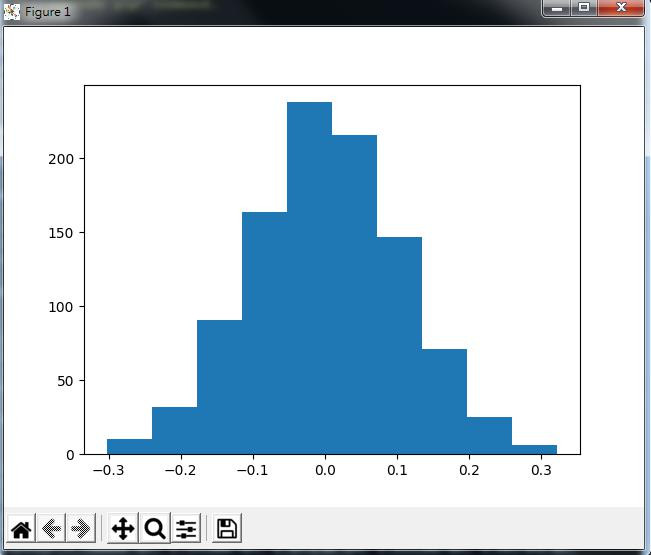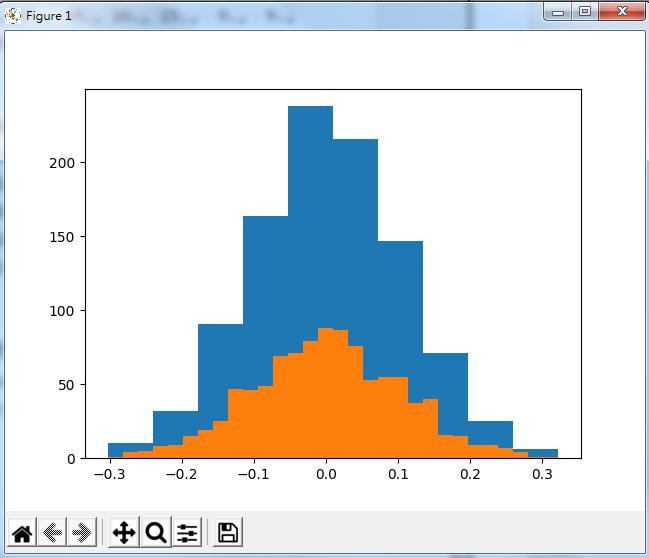``````>>> x = np.arange(1, 9, 2)
>>> y = 2 * x
>>> plt.plot(x, y, linewidth=1.5) # 這樣應該是y=2x的樣子
[<matplotlib.lines.Line2D object at 0x000000000BCCB220>]
>>> plt.show()
``````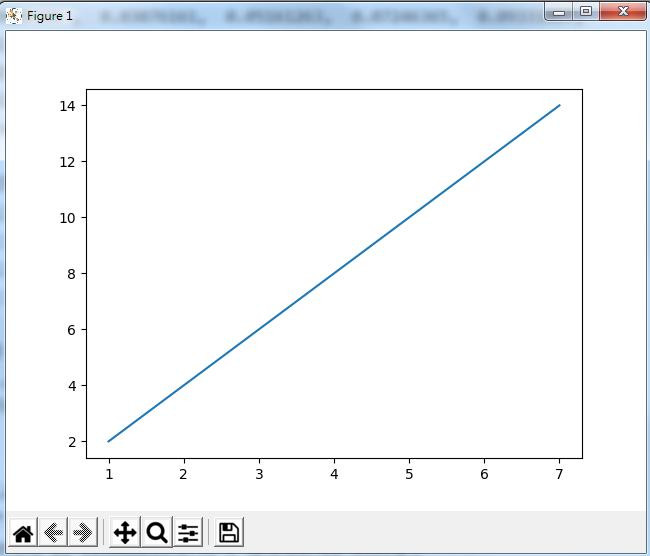``````>>> plt.plot([1,2,3,4])
>>> plt.show()
>>> plt.plot([1,2,3,4], [1,2,3,4]) # 留意X座標的變化!
>>> plt.show()
``````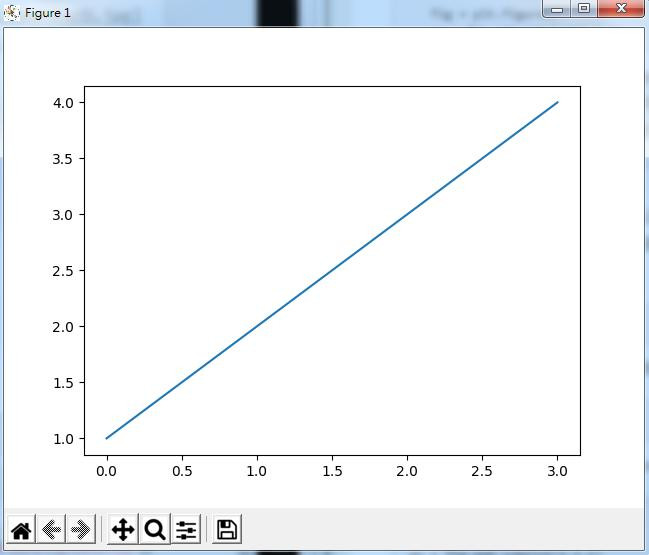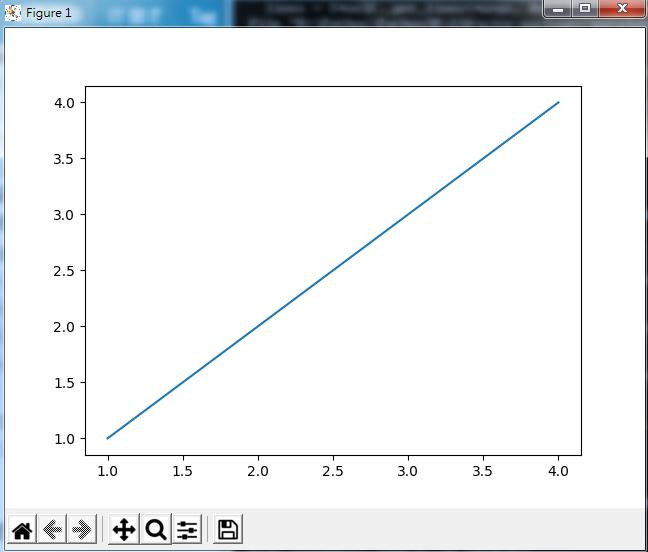figure的軸則是axes，可以用add_subplot(1,1,1)方法取用到，

(這不是很好解釋，如果一次只畫一張圖的話就不管它啦！)

``````>>> fig = plt.figure()
>>> ax = fig.add_subplot(1,1,1)
>>> ax.plot([1,2,3,4], [1,2,3,4])
[<matplotlib.lines.Line2D object at 0x000000000B540B80>]
>>> ax.set_title('Title') # 表題
Text(0.5, 1.0, 'Title')
>>> ax.set_xlabel("label: x") # X軸文字
Text(0.5, 0, 'label: x')
>>> ax.set_ylabel("label: y") # Y軸文字
Text(0, 0.5, 'label: y')
>>> fig.suptitle('Sup Title', fontsize=20, fontweight='bold') #
Text(0.5, 0.98, 'Sup Title')
``````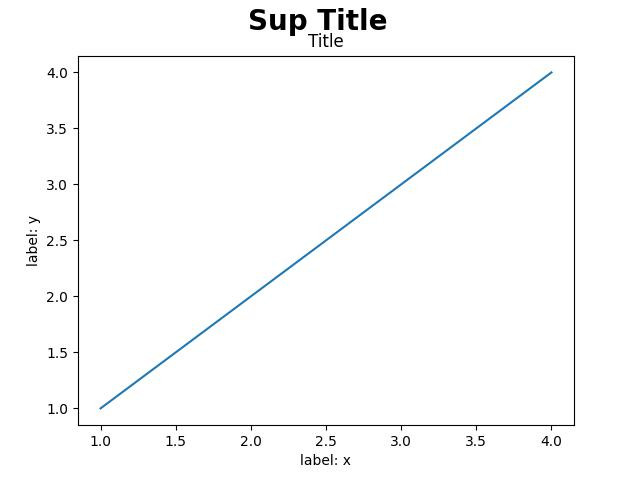``````# r代表紅色，x代表用'x'來表示點，且不畫線
>>> plt.plot([1,2,3,4], [3,5,15,18], 'rx')
[<matplotlib.lines.Line2D object at 0x000000000B856C10>]
# b代表藍色，'.'代表用單個小點表示一個點，'--'表示用虛線(dashed line)來畫線
>>> plt.plot([1,2,3,4], [3,9,1,6], 'b.--')
[<matplotlib.lines.Line2D object at 0x000000000B866310>]
# g代表綠色，o代表實心圓，linewidth表示粗度修為3
>>> plt.plot([1,2,3,4], [1,4,9,16], 'go-', linewidth=3)
[<matplotlib.lines.Line2D object at 0x000000000B856F40>]
>>> plt.legend(('red', 'blue', 'green'), loc='upper left') # 畫圖例及決定位置
<matplotlib.legend.Legend object at 0x000000000B757730>
>>> plt.grid(True) # 畫出網格
>>> plt.show()
``````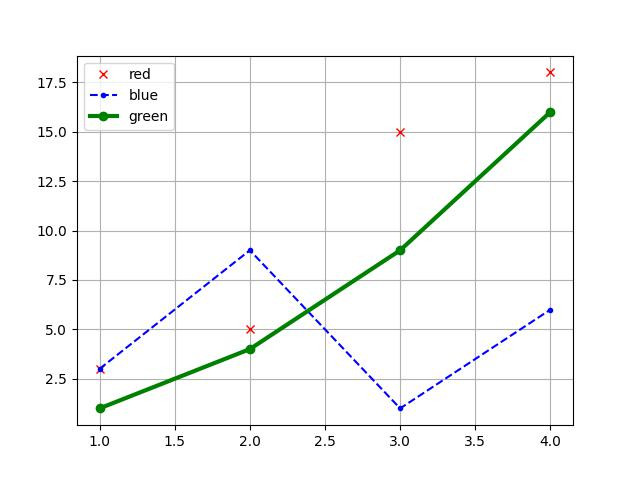``````>>> np.random.random(5)
array([0.22612501, 0.34256502, 0.21559228, 0.17675453, 0.1082928 ])
>>> x = np.random.random(500)
>>> y = np.random.random(500)
>>> plt.scatter(x, y)　# 其實用plt.plot(x, y, 'o')也可以啦！
<matplotlib.collections.PathCollection object at 0x000000000B566580>
>>> plt.show()
``````

``````>>> z = np.random.normal(0, 0.2, 500)
>>> fig = plt.figure()
>>> ax = plt.axes(projection='3d')
>>> ax.scatter3D(x, y, z, color='blue')
<mpl_toolkits.mplot3d.art3d.Path3DCollection object at 0x000000000C00A1F0>
>>> plt.show()
``````